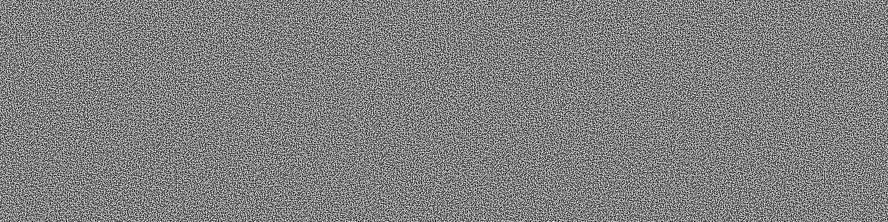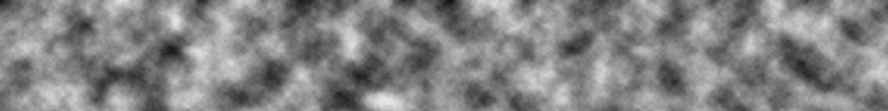Skip to content
{{ message }}

# joshforisha / open-simplex-noise-js

OpenSimplex noise for TypeScript/JavaScript

Switch branches/tags
Nothing to show

1474a72

## Files

Failed to load latest commit information.
Type
Name
Commit time

# OpenSimplex Noise

TypeScript implementation of OpenSimplex noise.

## Example

```import { makeNoise2D } from "open-simplex-noise";
// import { makeNoise2D } from "https://deno.land/x/open_simplex_noise/mod.ts"

const [width, height] = [888, 222];
const canvas = document.querySelector("canvas");
const ctx = canvas.getContext("2d");
const imageData = ctx.createImageData(width, height);
const noise2D = makeNoise2D(Date.now()); // Using current date as seed

for (let x = 0; x < width; x++) {
for (let y = 0; y < height; y++) {
const i = (x + y * width) * 4;
const value = (noise2D(x, y) + 1) * 128;
imageData.data[i] = value;
imageData.data[i + 1] = value;
imageData.data[i + 2] = value;
imageData.data[i + 3] = 255;
}
}
ctx.putImageData(imageData, 0, 0);```## Fractal Noise

For fractal noise results, which typically involves scaling frequencies and stacking octaves, see joshforisha/fractal-noise-js (NPM: fractal-noise). The functions there can be used with this library's noise algorithm to obtain varied results like the following:## API

```type Noise2D = (x: number, y: number) => number
type Noise3D = (x: number, y: number, z: number) => number
type Noise4D = (x: number, y: number, z: number, w: number) => number```

#### `makeNoise2D (seed: number) => Noise2D`

Initializes and returns a function to generate 2D noise.

#### `makeNoise3D (seed: number) => Noise3D`

Initializes and returns a function to generate 3D noise.

#### `makeNoise4D (seed: number) => Noise4D`

Initializes and returns a function to generate 4D noise.

## About

OpenSimplex noise for TypeScript/JavaScript

## Releases 18

Version 2.5.0 Latest
Apr 15, 2021

## Packages 0

No packages published